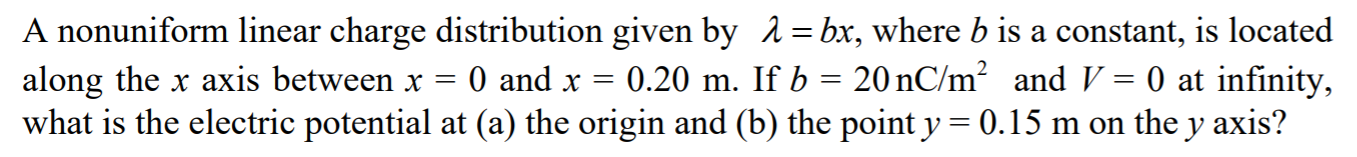Home / Answered Questions / Other / a-nonuniform-linear-charge-distribution-given-by-a-bx-where-b-is-a-constant-is-located-along-the-x-a-aw155

# (Solved): A Nonuniform Linear Charge Distribution Given By A = Bx, Where B Is A Constant, Is Located Along The...A nonuniform linear charge distribution given by a = bx, where b is a constant, is located along the x axis between x = 0 and x = 0.20 m. If b = 20 nC/mÂ² and V = 0 at infinity, what is the electric potential at (a) the origin and (b) the point y = 0.15 m on the y axis?

We have an Answer from Expert